### Home > A2C > Chapter Ch11 > Lesson 11.2.3 > Problem11-77

11-77.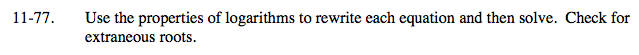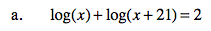log(x2 + 21x) = 2

x2 + 21x = 102

(x − 4)(x + 25) = 0

4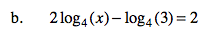$\text{log}_4\left(\frac{x^2}{3}\right)=2$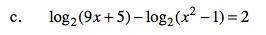$\text{log}_2 \left(\frac{9x+2}{x^{2}-1}\right)=2$

3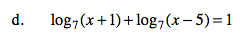See parts (a) and (b).